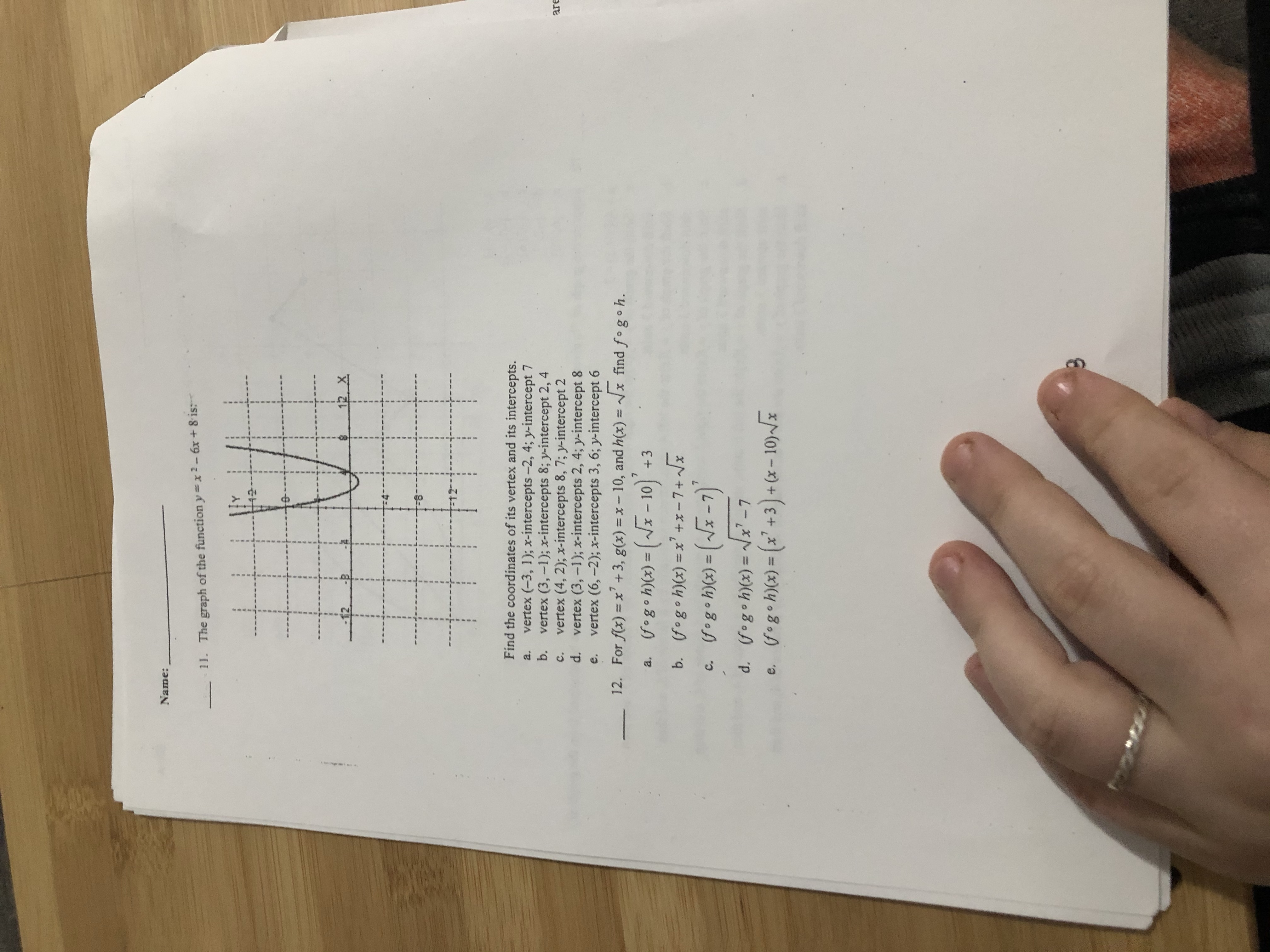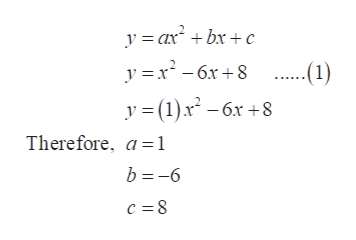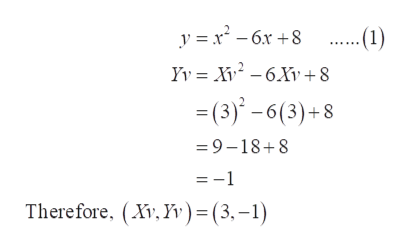# Name:-11. The graph of the function y=x2-6x+ 8 is:12 XFind the coordinates of its vertex and its intercepts.vertex (-3, 1); x-intercepts-2, 4; y-intercept 7b.a.vertex (3,-1); x-intercepts 8; y-intercept 2, 4vertex (4, 2); x-intercepts 8, 7; y-intercept 2d.arec.vertex (3, -1); x-intercepts 2, 4; y-intercept 8vertex (6,-2); x-intercepts 3, 6; y-intercept 6For f(x) = x' +3, g(x) = x- 10, and h(x) = x find fogoh.e.12.%3D%3D(fog•h)(x) = (~F - 10) +3(fogoh)(x) = x' +x-7+ /xgogoh)(x) = (~x -7)'a.b.%3DC.d. (fogoh)(x) = /x -7e. fogoh)(x) = (x' +3)+ (x – 10)~/x%3D%3D

Question
4 viewshelp_outlineImage TranscriptioncloseName: -11. The graph of the function y=x2-6x+ 8 is: 12 X Find the coordinates of its vertex and its intercepts. vertex (-3, 1); x-intercepts-2, 4; y-intercept 7 b. a. vertex (3,-1); x-intercepts 8; y-intercept 2, 4 vertex (4, 2); x-intercepts 8, 7; y-intercept 2 d. are c. vertex (3, -1); x-intercepts 2, 4; y-intercept 8 vertex (6,-2); x-intercepts 3, 6; y-intercept 6 For f(x) = x' +3, g(x) = x- 10, and h(x) = x find fogoh. e. 12. %3D %3D (fog•h)(x) = (~F - 10) +3 (fogoh)(x) = x' +x-7+ /x gogoh)(x) = (~x -7)' a. b. %3D C. d. (fogoh)(x) = /x -7 e. fogoh)(x) = (x' +3)+ (x – 10)~/x %3D %3D fullscreen
check_circle

Step 1

Option (d) is the correct option.

Consider the given function and the general equation form for the quadratic equation.

Compare the given function and the general equation form for the quadratic equation and find a, b and c.help_outlineImage Transcriptionclosey = ax + bx +c ..(1) y = (1).x² – 6x +8 y =x - 6x+8 Therefore, a=1 b =-6 fullscreen
Step 2

Then, find the value of x-coordinate of the vertex by the formula, Xv = -b/2a.

Step 3

Now, find the value of y-coordinate of the vertex by putting the valu...help_outlineImage Transcriptionclosey = x² - 6x +8 .(1) Yy = X - 6Xv +8 = (3)' – 6(3)+8 = 9-18+8 = -1 Therefore, (Xv, Yv)=(3,-1) fullscreen

### Want to see the full answer?

See Solution

#### Want to see this answer and more?

Solutions are written by subject experts who are available 24/7. Questions are typically answered within 1 hour.*

See Solution
*Response times may vary by subject and question.
Tagged in

### Calculus